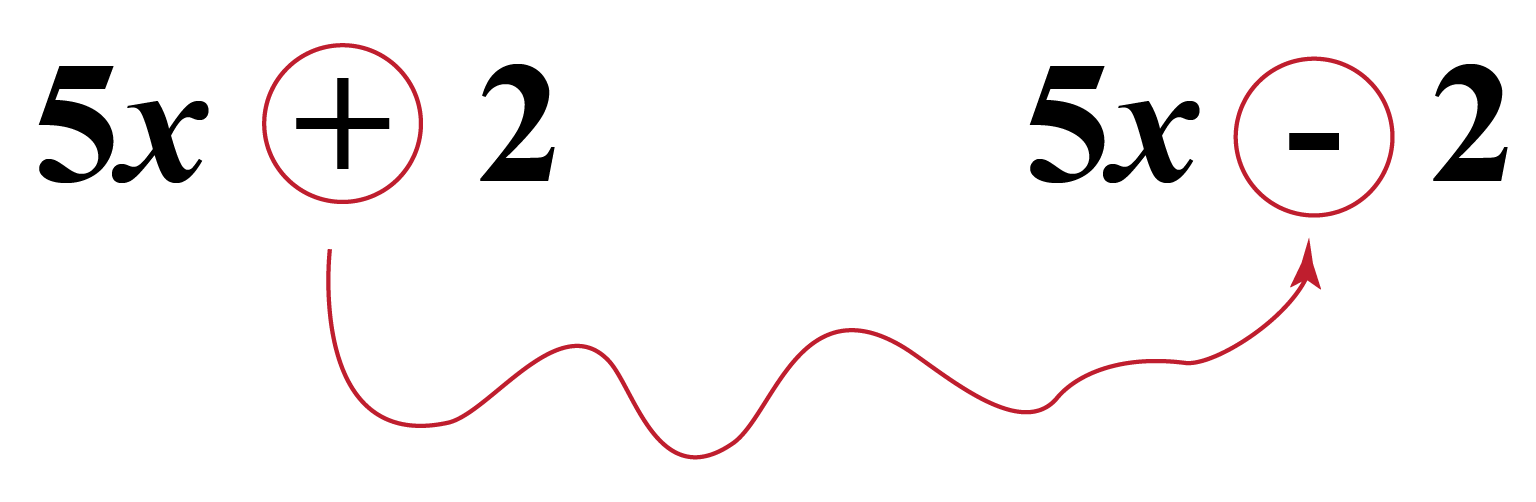# Conjugate in Math

Conjugate in Math

Hello kids! Do you know what conjugate means?

The word conjugate means a couple of objects that have been linked together. Except for one pair of characteristics that are actually opposed to each other, these two items are the same.## Lesson Plan

 1 What Is Meant by Conjugate in Math 2 Important Notes on Conjugate In Math 3 Solved Examples on Conjugate In  Math 4 Challenging Questions on Conjugate In Math 5 Interactive Questions on Conjugate In Math

## What Is Meant by Conjugate in Math?

Conjugate in math means to write the negative of the second term.

By flipping the sign between two terms in a binomial, a conjugate in math is formed.

The conjugate of $$a+b$$ can be written as $$a-b$$.

## How to Conjugate Binomials?

The conjugate of binomials can be found out by flipping the sign between two terms.

For example the conjugate of $$m+n$$ is $$m-n$$. In other words, it can be also said as $$m+n$$ is conjugate of $$m-n$$.

The conjugate of $$5x + 2$$ is $$5x - 2$$Look at the table given below of conjugate in math which shows a binomial and its conjugate.

 Binomial Conjugate of Binomial $$3 - \sqrt 2$$ $$3 + \sqrt 2$$ $$5 + \sqrt 7$$ $$5 - \sqrt 7$$ $$\sqrt 7 - \sqrt 2$$ $$\sqrt 7 + \sqrt 2$$ $$\sqrt 5 - 11$$ $$\sqrt 5 + 11$$ $$\sqrt 5 + \frac{1}{3}$$ $$\sqrt 5 - \frac{1}{3}$$

## How to Rationalize the Denominator Using Conjugates?

To rationalize the denominator using conjugate in math, there are certain steps to be followed.

Let us understand this by taking one example.

Example

Rationalize the denominator  $$\frac{1}{{5 - \sqrt 2 }}$$

Solution

Step 1: Find out the conjugate of the number which is to be rationalized. In our case that is $$5 + \sqrt 2$$

Step 2: Now multiply the conjugate, i.e.,  $$5 + \sqrt 2$$ to both numerator and denominator.

\begin{align} &= (\frac{1}{{5 - \sqrt 2 }}) \times (\frac{{5 + \sqrt 2 }}{{5 + \sqrt 2 }}) \\[0.2cm] &= \frac{{5 + \sqrt 2 }}{{(5 - \sqrt 2 )(5 + \sqrt 2 )}} \\[0.2cm] &= \frac{{5 + \sqrt 2 }}{{(5)^2 - (\sqrt 2 )^2}} \\[0.2cm] &= \frac{{5 + \sqrt 2 }}{{25 - 2}} \\[0.2cm] &= \frac{{5 + \sqrt 2 }}{{23}} \\ \end{align}

So this is how we can rationalize denominator using conjugate in math.

More Important Topics
Numbers
Algebra
Geometry
Measurement
Money
Data
Trigonometry
Calculus
More Important Topics
Numbers
Algebra
Geometry
Measurement
Money
Data
Trigonometry
Calculus